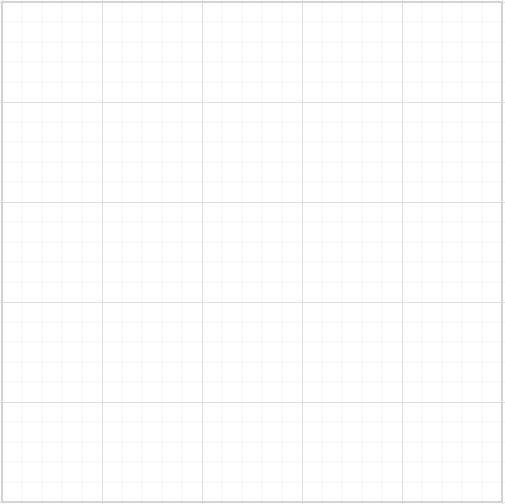# Model

The Diagram model represents the data to render the Diagram and to manipulate the Diagram elements. The following code illustrates how to define Diagram model.

• razor
• ``````//Creates diagram
<div>
@Html.EJ().Diagram("diagram").Width("100%").Height("100%").PageSettings(s=>s.PageWidth(2000).PageHeight(2000))
</div>``````To explore more model properties, refer to Model Properties.